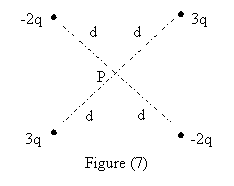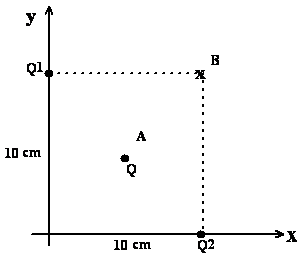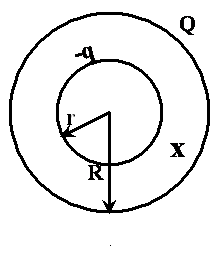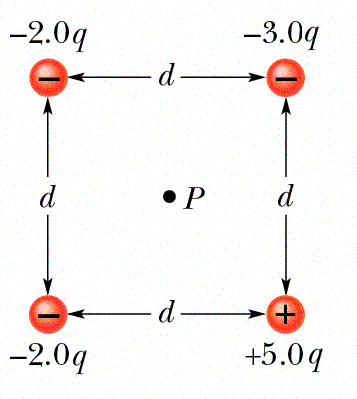KFUPM-Physics102-052
Dr.Aljalal
Sec16
Sec17
Sec18

Recitation ch 24
Answers at the end

Question 1
In figure (7), what is the net potential at point P due to the
four point charges if V = 0 at infinity ? [take d = 2 cm,
q = 1.0 micro-C].a   - 4.6*10**7 V.
b   4.6*10**7 V.
c   9.0*10**5 V.
d   - 9.0*10**5 V.
e   Zero.
==============================================
Question 2
Two conducting spheres A and B are electrically isolated.
The smaller sphere A has a total charge of 6*10**(-8) C
and radius R. Sphere B has a radius (2*R) and is neutral.
After connecting the two spheres by a conducting wire,
find the charge on sphere B.

a   - 6*10**(-8) Coulomb
b   + 2*10**(-8) Coulomb
c   + 4*10**(-8) Coulomb
d   + 6*10**(-8) Coulomb
e   + 3*10**(-8) Coulomb
==============================================
Question 3
In figure (4), an electron moves from point 'I' to point 'F'
in a uniform electric field directed as shown in the figure.a  The electron moves to a lower potential.
b  The electric field does positive work on the electron.
c  The electric field does negative work on the electron.
d  An external force is required to move the electron from I to F.
e  The electric potential energy of the electron increases.
==============================================
Question 4
Which one of the following statements is true?

a  We have to do work to move a charged particle along an
equipotential surface.
b  The electric field lines are perpendicular to the equipotential
surfaces.
c  The electric field is a scalar quantity.
d  The electric potential is a vector quantity.
e  Any two equipotential surfaces are always parallel.
==============================================
Question 5
In figure (6), Q1 = 2.0*10**(-6) C and Q2 = - 2.0*10**(-6) C.
What is the external work needed to move a charge
Q = - 4.0*10**(-6) C at constant speed from point A at the
center of the square to point B at the corner?a  - 7.2*10**(-6) Joule.
b   7.2*10**(-6) Joule.
c   5.1*10**(-6) Joule.
d   Zero.
e  - 5.1*10**(-6) Joule.
==============================================
Question 6
Two large, parallel, conducting plates are 20 cm apart and
have charges of equal magnitude but opposite signs on their
facing surfaces. An electron, placed anywhere between the two
plates experiences an electrostatic force of 1.6*10**(-15) N.
Find the magnitude of the potential difference between the
two plates.

a   4 kV
b   2 kV
c   50 kV
d   10 kV
e   30 kV
==============================================
Question 7
Which one of the following statements is CORRECT ?

a   All points of a conductor in electrostatic equilibrium
are at the same potential.
b   Electric field lines are always parallel to equipotential
surfaces.
c   The electric field at the surface of a conductor in
electrostatic equilibrium is parallel to the surface
of the conductor.
d   Electric field lines are always in the direction of
increasing electric potential.
e   If a conducting sphere carries a net charge, the
charge will be uniformly distributed over its volume.
==============================================
Question 8
In figure (5), a hollow sphere, of radius r that carries a
negative charge -q, is put inside another hollow sphere, of
radius R that carries a positive charge Q. At a distance x
from the common center, such that r < x < R, the electric
potential is:a   k*[(Q/R)-(q/x)].
b   k*[(Q/R)-(q/r)].
c   k*[(Q/R)+(q/x)].
d   k*[(Q/x)-(q/R)].
e   k*[(Q/R)+(q/r)].
==============================================
Question 9
The electric potential at point A in an electric field is
15 V smaller than at point B. If a charge q = -2.0 C is
moved from A to B, then the electric potential energy of
this charge will:

a   increase by 15 J.
b   increase by 25 J.
c   decrease by 30 J.
d   decrease by 15 J.
e   increase by 30 J.
==============================================
Question 10
The electric potential at points in the xy�plane is given by:
V = (x**3 - 2*x*y) Volts,
where x and y are in meters. The magnitude of the electric
field at the point with the coordinates x = 1 m and y = 2 m is:

a   Sqrt(3) V/m.
b   Zero.
c   Sqrt(5) V/m.
d   Sqrt(8) V/m.
e   Sqrt(2) V/m.
==============================================
Question 11
In figure 6, point P is at the center of the square.
Find the net electric potential at point P.
Assume V = 0 at infinity.a   + 2.8 kq/d
b   - 1.4 kq/d
c   zero
d   - 2.8 kq/d
e   + 1.4 kq/d
==============================================
Question 12
Two balls with charges 5.0 micro-C and 10 micro-C are at a
distance of 1.0 m from each other. In order to reduce the
distance between them to 0.5 m the amount of work to be
performed is:

a   4.5*10**(-4) J.
b   1.2*10**(-4) J.
c   45.0 J.
d   0.23 J.
e   0.45 J.
==============================================
Question 13
Two positive charges, each of magnitude Q, are fixed at two
corners of an equilateral triangle (see figure 5). The work
required, by an external agent, to move a third positive
charge q from A to C is:a   - 2kQq/d
b   + kQq/d
c   + 2kQq/d
d   - kQq/d
e   zero
==============================================
Question 14
Find the electric potential at the center of a charged
metal sphere of radius 15 cm if the electric field at its
surface is 1.2*10**4 N/C.

a   1.8 kV
b   2.7 kV
c   zero
d   1.2 kV
e   Infinity
==============================================
Question 15
What is the external work required to bring four 2.0*10**(-9) C
point charges from infinity and to place them at the corner of
a square of side 0.14 m

a  1.8*10**(-6) Joule.
b  0.6*10**(-6) Joule.
c  0.3*10**(-6) Joule.
d  1.0*10**(-6) Joule.
e  1.4*10**(-6) Joule.
==============================================
Question 16
Over a certain region, the electric potential is given as
V = (3.0*(x**2)*y)+(y**2)+(y*z) (volts).
Find the magnitude of the electric field at the point
(1.0,1.0,1.0), where all distances are in meters.

a   3.6 N/C
b   2.4 N/C
c   8.5 N/C
d   1.0 N/C
e   zero
==============================================
Question 17
Two electrons are fixed 2.0 cm apart. Another electron is
shot from infinity with a speed v and comes to rest at a
point midway between the two electrons. Find v.

a   742 m/s
b   318 m/s
c   255 m/s
d   612 m/s
e   963 m/s
==============================================

1     c
2     c
3     b
4     b
5     d
6     b
7     a
8     a
9     c
10     c
11     d
12     e
13     c
14     a
15     e
16     c
17     b